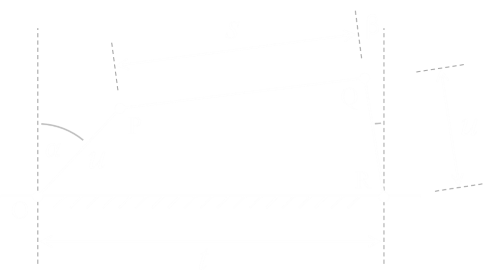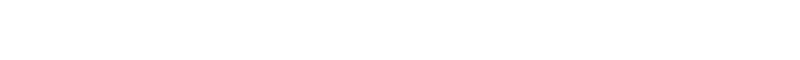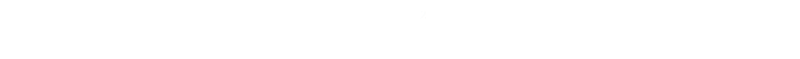﻿ Freudenstein’s Equation# Freudenstein’s EquationLast week, I wrote about the basic principles of Ackerman Steering. In that article I hinted that the formula describing the angles of the wheels was non-trivial. Rather than leave that statement hanging, I thought I’d dive into the math in this article.

With four fixed links, and four pivots, the relationship is described by a form of the Freudenstein’s Equation.

The geometry of Ackerman steering is symmetrical, so in the neutral position, the rods form a trapezium, and this makes the analysis a little simpler. Here is a diagram at a generic position:t is the track width. s is the length of the tie-rod. u is the length of both arms. α is the angle for the left wheel. β is the angle for the right wheel

Our goal is to derive a formula for the angle β, given the angle α, and the lengths (ie. If we know the angle of one wheel, can we determine the angle of the other).

If we define position O as the origin, it's possible to work out the coordinates for points P and Q.The tie rod is fixed, length s, and we can derive another equation for it based on Pythagoras and the points P and Q:Substituting in the coordinates we get:Grouping together similar terms to simplify:Using the trig identity that sin2A + cos2A = 1, this simplifies to:Rearranging his into constant terms, and terms of cosβ and sinβ:This result is the Freudenstein Equation:Where the constants: K1, K2 and K3 are:### Solving

This equation can be elegantly solved by using the half-angle tangent trig identities:Using these half angle formulas on the Freudenstein equation, we can group the tangent terms to form a quadratic:The trusty quadratic formula can be applied:And here we get our final result; A way to derive the angle β using the other wheel angle α, and the lengths of the linkages:The quadtratic formula gives two roots ± as there are, potentially, two answers (one where the rods cross, and one where they do not).

### Simulation

Below is a small interactive simulation of the steering system. Moving the mouse (or dragging on the tablet) left and right, simulated turning the steering wheel right or left. You can see that 'inside' wheel turning at a different rate to the 'outside' wheel.

Using the six buttons on the left it is possible to adjust the geometery (length) of the various roads. To the right of the link adjusting buttons is a toggle to render the arc of the tie rods; this helps visualize what is going on. As the the trapezium is deformed, the rods trace a circular arc around the wheel pivot. The next button toggles rendering of the wheels and, finally, the end button resets the lengths to a default demonstration position.

You can find a complete list of all the articles here.Click here to receive email alerts on new articles.